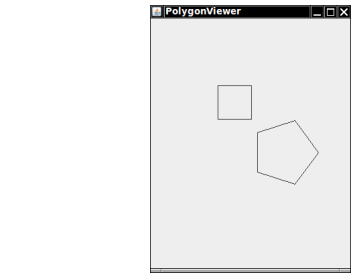Implement a class Polygon that contains an array list of Point2D.Double objects. Support methods

public void draw(Graphics2D g2)

Draw the polygon by joining adjacent points with a line, and then closing it up by joining the end and start points. Write a graphical application that draws a square and a pentagon using two Polygon objects.

Here is a sample program output:Complete the following file:

### Polygon.java

Use the following files:

PolygonComponent.java

```import javax.swing.JComponent;
import java.awt.Graphics;
import java.awt.Graphics2D;
import java.awt.geom.Point2D;

/**
Displays two polygons.
*/
public class PolygonComponent extends JComponent
{
public void paintComponent(Graphics g)
{
Graphics2D g2 = (Graphics2D) g;

Polygon square = new Polygon();

square.draw(g2);

Polygon pentagon = new Polygon();
double centerX = 200;
double centerY = 200;
for (int i = 0; i < 5; i++)
{
double angle = 2 * Math.PI * i / 5;
}

pentagon.draw(g2);
}
}
```

PolygonViewer.java

```import javax.swing.*;

public class PolygonViewer
{
public static void main(String[] args)
{
JFrame frame = new JFrame();

final int FRAME_WIDTH = 300;
final int FRAME_HEIGHT = 400;

frame.setSize(FRAME_WIDTH, FRAME_HEIGHT);
frame.setTitle("PolygonViewer");
frame.setDefaultCloseOperation(JFrame.EXIT_ON_CLOSE);

PolygonComponent component = new PolygonComponent();